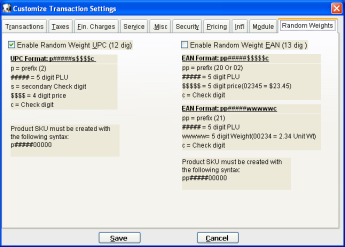Figure 3-14b

The Random Weights feature enables use and setup of composite barcodes, which can be used in conjunction with scales that print barcode labels with specific SKU and price/weight information.

Enable Random Weight UPC (12 digits)
The checkbox here allows you to toggle this feature on/off. The 12-digit composite UPC can be used with barcode labels that have already calculated the price, using the following syntax and steps:

1. Create a default 12 digit SKU in MicroBiz. The price entered on this SKU should be the price per unit (for example \$2.00 if \$2.00 per pound):
• Syntax: p#####00000, Example: 20000100000.
• In the example above, 2= check digit, 00001 = PLU code, 00000 = (syntax which must be added to work).
2. You will need a scale which will calculate the price based on weight measurement. Here is an example of what the barcode format which the scale will print out:
• Syntax: p#####s\$\$\$\$c, Example: 200001807541
• In the example above, 2= Prefix, 00001= PLU Code, 8= Secondary Check Digit, 0754 = Price (\$7.54 in this example), 1= Check Digit.
3. Keying or scanning this barcode will pull up the SKU as entered in MicroBiz (20000100000) with the assigned price as printed out by the barcode provided by the scale (\$7.54 in the example). MicroBiz will calculate the weight (QTY) based on the SKU and extended price (3.77 pounds using our example above).

Enable Random Weight EAN (13 digits)
This checkbox toggles the EAN composite barcodes on/off. The 13-digit composite EAN can be used with barcode labels that have already calculated the price or weight, using the following syntax and steps:
Barcode if printed by Price:

1. Create a default 13 digit SKU in MicroBiz. The price entered on this SKU should be the price per unit (for example \$2.00 if \$2.00 per pound):
• Syntax: pp######\$\$\$\$\$c, Example: 020000200000
• In the example aboce, 02= Prefix, 000002 = PLU Code, 00000 = (syntax which must be added to work).
2. You will need a scale which will calculate the price based on weight measurement. Here is an example of what the barcode format which the scale will print out:
• Syntax: pp#####\$\$\$\$\$c, Example: 0200002023451
• In the example above, 02 = Prefix, 000002 = PLU Code, 02345 = Price (\$23.45 in this example), 1 = Check Digit.
3. Keying or scanning this barcode will pull up the SKU as entered in MicroBiz (020000200000) with the assigned price as printed out by the barcode provided by the scale (\$23.45 in the example).

Barcode if printed by Weight:

1. Create a default 12 digit SKU in MicroBiz. The price entered on this SKU should be the price per unit (for example \$2.00 if \$2.00 per pound). The price entered here is important because MicroBiz will calculate the price based on the unit measurement calculated and printed on the barcode by the scale:
• Syntax: pp#####00000, Example: 210000300000
• In the example above, 21= Prefix, 00003 = PLU Code, 00000 = (syntax which must be added to work).
2. You will need a scale which will calculate the weight. Here is an example of what the barcode format, which the scale will print out:
• Syntax: pp#####wwwwwc, Example: 2100003002341
• In the example above, 21= Prefix, 00003 = PLU Code, 00234 = Unit Measurement (2.34 units in the example), 1 = Check Digit.
3. Keying or scanning this barcode will pull up the SKU as entered in MicroBiz (210000300000) with the assigned units as provided by the scale barcode (2.34 units in the example). MicroBiz will calculate the price by multiplying the amount of units by the price entered for the product in "Add/Edit Inventory."